# College Chemistry : Thermodynamics

## Example Questions

← Previous 1

### Example Question #1 : Thermodynamics

The second law of thermodynamics states which of the following is true regarding an isolated system?

Two systems in thermal equilibrium with a third system are in thermal equilibrium with each other

The entropy approaches a constant value as the temperature of the system approaches zero Kelvin

The entropy can only decrease

Energy in the form of heat is always conserved

The entropy can only increase

The entropy can only increase

Explanation:

The entropy cannot decrease in an isolated system because the energy can only be degraded. Since the system is isolated, no higher-grade energy—or any energy at all—is being introduced into the system. As a result, the entropy cannot decrease. The other answer choices relate to the other laws of thermodynamics.

### Example Question #1 : Laws Of Thermodynamics

Which of the following statements is true of standard states?

Solute in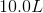of solution

Gas at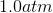pressure

Temperature at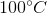Gas at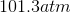Solute of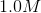concentration

Gas atpressure

Explanation:

Standard states are defined as a specific set of conditions, such as when a gas is atconcentration, and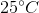.

Standard enthalpy of formation, the energy required for form 1 mole of a compound from its constituent elements, occurs when elements are in their standard states.

### Example Question #3 : Thermodynamics

How much heat is required to raise the temperature of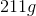of water from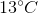to?  (Specific heat capacity of water is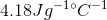)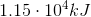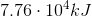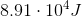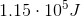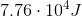Explanation: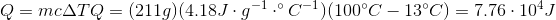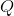is positive because heat flows into the system to raise the temperature of the water.

### Example Question #4 : Thermodynamics

How much heat is required to raise the temperature of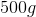of water from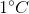to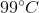? Specific heat capacity of water is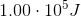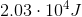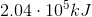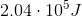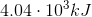Explanation: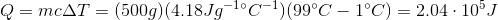### Example Question #5 : Thermodynamics

"In a natural thermodynamic process, the sum of the entropies of the interacting systems increases." Which law of thermodynamics does this statement refer to?

Zeroth law of thermodynamics

First law of thermodynamics

Second law of thermodynamics

Third law of thermodynamics

Second law of thermodynamics

Explanation:

There are four main laws of thermodynamics, which describe how temperature, energy, and entropy behave under various circumstances. The zeroth law of thermodynamics helps to define temperature; it states that if two systems are each in thermal equilibrium with a third system, they must be in thermal equilibrium with each other. The first law of thermodynamics negates the possibility of perpetual motion; it states that when energy passes into or out of a system, the system's internal energy changes in accord with the law of conservation of energy. The second law of thermodynamics also negates the possibility of perpetual motion; it states that in a natural thermodynamic process, the sum of the entropies of the interacting systems increases. Lastly, the third law of thermodynamics states that the entropy of a system approaches a constant value as the temperature nears absolute zero.

### Example Question #6 : Thermodynamics

Calculating heat

How much heat is absorbed by a copper penny as it warms from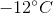to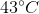assuming the penny is pure copper with a mass of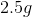?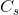of copper is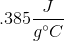.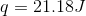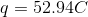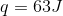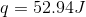Explanation:

Use the equation that relates heat, mass, specific heat, and change in temperature: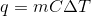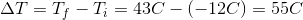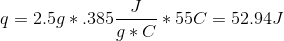### Example Question #7 : Thermodynamics

How do we know if a reaction is spontaneous?

The sign of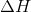The value of(the rate constant) tells us

The second law of thermodynamics

The first law of thermodynamics

The sign of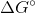The sign ofExplanation:

A reaction is spontaneous if and only if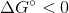. The sign ofonly tells us if a reaction is exothermic or endothermic. The rate constant only tells us the rate at which a given chemical reaction proceeds based on the reactants and the products. The first law of thermodynamics only tells us that energy is conserved, and can neither be created nor destroyed. The second law of thermodynamics states that the entropy of the universe is spontaneously increasing, however, under the right conditions, these reactions may proceed.

### Example Question #8 : Thermodynamics

What is the standard change in free energy for a reaction run at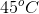with an equilibrium constant of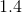?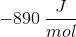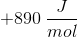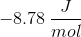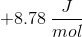Explanation:

In this question, we're told of a reaction that is run at a given temperature and with a certain equilibrium constant. We're asked to evaluate the standard change in free energy for this reaction.

First, right off the bat, we can see that the equilibrium constant for the reaction is greater than one. As a result, we know that under standard conditions, equilibrium of this reaction will favor products. Hence, we know right away that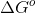will be negative, which allows us to rule out any answer choices that are positive.

To actually calculate the value of, we'll need to use an equation that relates this quantity with temperature and the equilibrium constant. We can do so by using the following expression.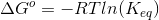If we plug in the values that we know, we can solve for the correct answer.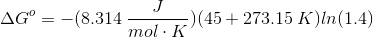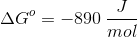### Example Question #9 : Thermodynamics

Calculate the change in free energy, in kilojoules, of the reaction at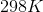by using the free energies of formation.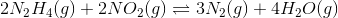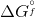values, in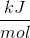, for molecules are as follows: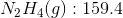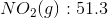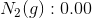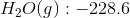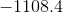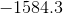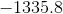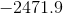Explanation:

Recall how to find the change in free energy for a reaction from using free energies of formation: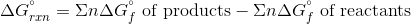Whereis the number of moles of the molecule.

Plug in the given values to find the change in free energy for the reaction.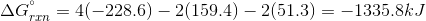### Example Question #10 : Thermodynamics

Suppose that a given chemical reaction has an enthalpy change of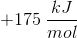and an entropy change of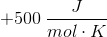. At what temperature would this chemical reaction be in equilibrium?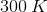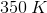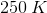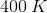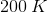Explanation:

For this question, we're asked to determine the temperature needed for a reaction to be in a state of equilibrium. We are also provided with the entropy and enthalpy changes for this reaction.

Remember that for a reaction to be in a state of equilibrium, the value for its change in free energy must be equal to zero. Furthermore, we can state the relationship between the change in free energy with the change in entropy, the change in enthalpy, and the temperature as follows.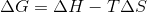Because the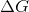term in the above expression must be equal to zero, we can plug this value in and then isolate the term for temperature.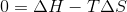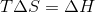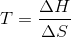Now that we have this expression, we just need to plug in the two values given to us in the question stem. Remember to make the units match though!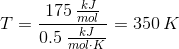← Previous 1